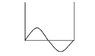# Quantum mechanics - particle in a box

• stevebrown

#### stevebrown

SOLVED: Quantum mechanics - particle in a box

## Homework Statement

We have a box with an electron in it and we know for sure that the electron is in the left half of the box. What is the probability that the electron is found in the ground state if we perform a measure on it?

## The Attempt at a Solution

A class mate told me that the probability is zero since it's impossible to measure/calculate the probability because the probability is time-dependent and the time-dependency is eliminated here.

Is that really true? And if it is, why is the time-dependency eliminated?

I'm totally lost here... don't even know where to begin looking for a correct answer so any hint is very appreciated.

Best regards,
Steve

Last edited:

This is what i think but i may be wrong but here goes...

if the electron is in the left side of the box for sure then the electron must be in some excited state. Excited state because excited states will have probability densities that are antisymmetric. The solutions for square well form a basis for space so they can be written as a superposition of other solutions in Fourier Series. The Fourier coefficients represent probabilities of the measurement of the corresponding energy. That is

$$f(x) = \sum_{n} c_{n} f_{n}(x)$$
Cn represents the probability of measuring the particle in state fn with energy of fn.

This is what i think but i may be wrong but here goes...

if the electron is in the left side of the box for sure then the electron must be in some excited state. Excited state because excited states will have probability densities that are antisymmetric. The solutions for square well form a basis for space so they can be written as a superposition of other solutions in Fourier Series. The Fourier coefficients represent probabilities of the measurement of the corresponding energy. That is

$$f(x) = \sum_{n} c_{n} f_{n}(x)$$
Cn represents the probability of measuring the particle in state fn with energy of fn.

There is a nonzero probability of being in the ground state. I am not sure why you concluded that it must be an excited state. The wavefunction of the particle has no definite parity (with respect to the center of the well) so no conclusion can be made from parity considerations. The overlap with the ground state is not zero (assuming that the wavefunction is a constant for 0<x<a/2, which is not clear from the question)

## Homework Statement

We have a box with an electron in it and we know for sure that the electron is in the left half of the box. What is the probability that the electron is found in the ground state if we perform a measure on it?
The question is ambiguous. I *assume* that they mean that it is equally likely to be found anywhere in the left half in which case the wavefunction is given by psi(x) = C for 0<x<a/2 and zero for x>a/2. Normalize the wavefunction which will give you the value of the constant C. Then determine the overlap of that wavefunction with the ground state, i.e. calculate
$$\int_0^a dx \psi_{ground ~state}^* ~~\psi(x)$$
The magnitude squared of this gives the probability that the particle be found in the ground state.

## The Attempt at a Solution

A class mate told me that the probability is zero since it's impossible to measure/calculate the probability because the probability is time-dependent and the time-dependency is eliminated here.

Is that really true? And if it is, why is the time-dependency eliminated?

I'm totally lost here... don't even know where to begin looking for a correct answer so any hint is very appreciated.

Best regards,
Steve
It's true that this state will not stay like this as time passes. But the question makes sense if it is asking what's the probability of observing the particle in th eground state at t=0.

Thanks for the help! I get it now.

There is a nonzero probability of being in the ground state. I am not sure why you concluded that it must be an excited state. The wavefunction of the particle has no definite parity (with respect to the center of the well) so no conclusion can be made from parity considerations. The overlap with the ground state is not zero (assuming that the wavefunction is a constant for 0<x<a/2, which is not clear from the question)

the reason i concluded that the wave function has parity because i was under the impression that the probability density of the wavefunction in a square well is anti symmetric or symmetric given the state.

Doesn't one of the excited state wavefunctions look like the diagram??

#### Attachments

•1.JPG
4.2 KB · Views: 365
the reason i concluded that the wave function has parity because i was under the impression that the probability density of the wavefunction in a square well is anti symmetric or symmetric given the state.

Doesn't one of the excited state wavefunctions look like the diagram??

The solutions of the time independent Schrodinger equation do have definite parity with respect to the center of the well, yes. But the actual wavefunction of a particle does not have to be of any definite parity! I never implied that the stationary states had no definite parity, I only said that the wavefunction given in the above problem had no definite parity.